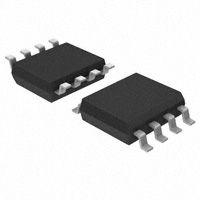< id="6e2kg">< id="6e2kg">< id="6e2kg">< id="6e2kg">< id="6e2kg">< id="6e2kg">< id="6e2kg">< id="6e2kg">< id="6e2kg">< id="6e2kg">< id="6e2kg">< id="6e2kg">< id="6e2kg">< id="6e2kg">< id="6e2kg">< id="6e2kg">< id="6e2kg">< id="6e2kg">< id="6e2kg">< id="6e2kg">< id="6e2kg">< id="6e2kg">< id="6e2kg">< id="6e2kg">< id="6e2kg">< id="6e2kg">< id="6e2kg">< id="6e2kg">< id="6e2kg">< id="6e2kg">< id="6e2kg">< id="6e2kg">< id="6e2kg">< id="6e2kg">< id="6e2kg">< id="6e2kg">< id="6e2kg">< id="6e2kg">< id="6e2kg">< id="6e2kg">< id="6e2kg">< id="6e2kg">< id="6e2kg">< id="6e2kg">< id="6e2kg">< id="6e2kg">< id="6e2kg">< id="6e2kg">< id="6e2kg">< id="6e2kg"> < id="6e2kg">< id="6e2kg">< id="6e2kg">< id="6e2kg">< id="6e2kg">< id="6e2kg">< id="6e2kg">< id="6e2kg">< id="6e2kg">< id="6e2kg">< id="6e2kg">< id="6e2kg">< id="6e2kg">< id="6e2kg">< id="6e2kg">< id="6e2kg">< id="6e2kg">< id="6e2kg">< id="6e2kg">< id="6e2kg">< id="6e2kg">< id="6e2kg">< id="6e2kg">< id="6e2kg">< id="6e2kg">< id="6e2kg">< id="6e2kg">< id="6e2kg">< id="6e2kg">< id="6e2kg">< id="6e2kg">< id="6e2kg">< id="6e2kg">< id="6e2kg">< id="6e2kg">< id="6e2kg">< id="6e2kg">< id="6e2kg">< id="6e2kg">< id="6e2kg">< id="6e2kg">< id="6e2kg">< id="6e2kg">< id="6e2kg">< id="6e2kg">< id="6e2kg">< id="6e2kg">< id="6e2kg">< id="6e2kg">< id="6e2kg"> < id="6e2kg">< id="6e2kg">< id="6e2kg">< id="6e2kg">< id="6e2kg">< id="6e2kg">< id="6e2kg">< id="6e2kg">< id="6e2kg">< id="6e2kg">< id="6e2kg">< id="6e2kg">< id="6e2kg">< id="6e2kg">< id="6e2kg">< id="6e2kg">< id="6e2kg">< id="6e2kg">< id="6e2kg">< id="6e2kg">< id="6e2kg">< id="6e2kg">< id="6e2kg">< id="6e2kg">< id="6e2kg">< id="6e2kg">< id="6e2kg">< id="6e2kg">< id="6e2kg">< id="6e2kg">< id="6e2kg">< id="6e2kg">< id="6e2kg">< id="6e2kg">< id="6e2kg">< id="6e2kg">< id="6e2kg">< id="6e2kg">< id="6e2kg">< id="6e2kg">< id="6e2kg">< id="6e2kg">< id="6e2kg">< id="6e2kg">< id="6e2kg">< id="6e2kg">< id="6e2kg">< id="6e2kg">< id="6e2kg">< id="6e2kg">型号： ACS712ELCTR-05B-T 厂商：Allegro MicroSystems, LLC 标准：分类： 传感器，变送器 ， 电流变送器 描述： sensor current 5A 5V BI 8-soic PDF： 预览 报错 收藏 赞

Datasheet下载地址

 本地下载 >> 厂商下载 >>

ACS712ELCTR-05B-T的详细信息

Datasheets： Performance and Efficiency Applications 3,000 Sensors, Transducers Current Transducers - Tape & Reel (TR) AC/DC Hall Effect, Open Loop 5A 1 Ratiometric, Voltage 185mV/A DC ~ 80kHz ±1.5% ±1.5% 4.5 V ~ 5.5 V 5μs 13mA -40°C ~ 85°C Bidirectional Surface Mount 8-SOIC (0.154", 3.90mm Width) ACS712 620-1431-ND - BOARD EVAL FOR ASEK712ELC-05B 620-1189-2ACS712ELCTR05BT
ACS712
Fully Integrated, Hall Effect-Based Linear Current Sensor IC
with 2.1 kVRMS Isolation and a Low-Resistance Current Conductor
Features and Benefits
Description
The Allegro? ACS712 provides economical and precise
solutionsforACorDCcurrentsensinginindustrial, commercial,
and communications systems. The device package allows for
easy implementation by the customer. Typical applications
mode power supplies, and overcurrent fault protection. The
device is not intended for automotive applications.
?
?
?
?
?
?
?
?
?
?
?
?
?
?
?
Low-noise analog signal path
Device bandwidth is set via the new FILTER pin
5 μs output rise time in response to step input current
80 kHz bandwidth
Total output error 1.5% at TA= 25°C
Small footprint, low-profile SOIC8 package
1.2 mΩ internal conductor resistance
2.1 kVRMS minimum isolation voltage from pins 1-4 to pins 5-8
5.0 V, single supply operation
66 to 185 mV/A output sensitivity
Output voltage proportional to AC or DC currents
Factory-trimmed for accuracy
Extremely stable output offset voltage
Nearly zero magnetic hysteresis
Ratiometric output from supply voltage
The device consists of a precise, low-offset, linear Hall circuit
with a copper conduction path located near the surface of the
die. Applied current flowing through this copper conduction
pathgeneratesamagneticfieldwhichtheHallICconvertsintoa
proportionalvoltage.Deviceaccuracyisoptimizedthroughthe
close proximity of the magnetic signal to the Hall transducer.
A precise, proportional voltage is provided by the low-offset,
chopper-stabilized BiCMOS Hall IC, which is programmed
for accuracy after packaging.
TüV America
Certificate Number:
U8V 06 05 54214 010
The output of the device has a positive slope (>VIOUT(Q)
)
when an increasing current flows through the primary copper
conduction path (from pins 1 and 2, to pins 3 and 4), which is
the path used for current sampling. The internal resistance of
this conductive path is 1.2 mΩ typical, providing low power
loss. The thickness of the copper conductor allows survival of
Package: 8 Lead SOIC (suffix LC)
Continued on the next page…
Approximate Scale 1:1
Typical Application
+5 V
8
7
1
2
VCC
IP+
IP+
VOUT
C
VIOUT
0.B1YPμF
IP
ACS712
6
5
3
4
FILTER
IP–
IP–
C
1FnF
GND
Application 1. The ACS712 outputs an analog signal, VOUT
that varies linearly with the uni- or bi-directional AC or DC
primary sampled current, IP, within the range specified. CF
is recommended for noise management, with values that
depend on the application.
.
ACS712-DS, Rev. 15